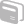# Activities

••• ##### Subject Area

• Math: Geometry: Quadrilaterals and Polygons

• ##### Author9-12

50 Minutes

• ##### Device
• TI-89 / TI-89 Titanium

## Finding the Area of Regular Polygons

#### Activity Overview

Students discover a very intuitive method for determining the area of a regular polygon. They use that information to develop the standard area formula.

This Activity comes from SIMMS Integrated Mathematics Level 1, © Kendall/Hunt Publishing Company.

#### Before the Activity

Print the PolygonArea.SE.pdf file for each student.

Print a teacher copy of the PolygonArea.TE.pdf file, as it provides sample answers to the questions posed.

#### During the Activity

In the Exploration students will use the TI-89 calculator and The Geometer's Sketchpad® App to construct regular polygons, determine their area in two different manners, and use that information to write a formula for the area of a regular polygon.

In the Discussion students will examine the standard formula for the area of a regular polygon and determine if it is equivalent to the one they developed in the Exploration.

The Exploration can be done individually or in pairs. The Discussion can be done individually, in small groups, or can be assigned as homework.

A whole class discussion of student responses is helpful to ensure students fully understand the mathematics involved.

#### After the Activity

Review student results:

• As a class, discuss questions that appeared to be more challenging
• Re-teach concepts as necessary